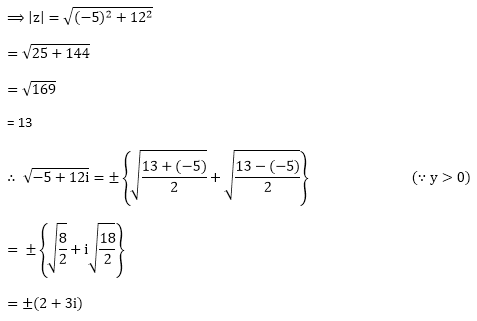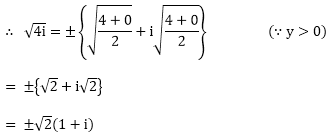# Chapter 13: Complex Numbers – Exercise 13.3

## Complex Numbers – Exercise 13.3 – Q.1(i)

Let z = – 5 + 12i## Complex Numbers – Exercise 13.3 – Q.1(ii)

Let z = – 7 – 24i## Complex Numbers – Exercise 13.3 – Q.1(iii)

Let z = 1 – i## Complex Numbers – Exercise 13.3 – Q.1(iv)

Let z = – 8 – 6i## Complex Numbers – Exercise 13.3 – Q.1(v)

Let z = 8 – 15i## Complex Numbers – Exercise 13.3 – Q.1(vi)## Complex Numbers – Exercise 13.3 – Q.1(vii)## Complex Numbers – Exercise 13.3 – Q.1(viii)

Let z = 4i

then |z| = |4i|

= 4|i|     (∵ |z1 z2 | = |z1 |×|z2|)

= 4        (∵ | i | = 1)## Complex Numbers – Exercise 13.3 – Q.1(ix)

Let z = – i

then |z| = | – i |

= | – 1| × |i|         (∵ |z1 z2 | = |z1| × |z2|)

= 1 × i                  (∵ | i | = 1)### Course Features

• Video Lectures
• Revision Notes
• Previous Year Papers
• Mind Map
• Study Planner
• NCERT Solutions
• Discussion Forum
• Test paper with Video Solution